# Advanced Tutorial Lesson 2: Designing Active Sallen-Key Filters

 Tutorial Project: Designing Active Sallen-Key FiltersObjective: In this project, you will use an operational amplifier to build active lowpass, highpass and bandpass filters. Concepts/Features: Operational Amplifier Active Filter Lowpass Filter Highpass Filter Bandpass Filter AC Frequency Sweep Custom Plot Multistage Filter Cascaded Filter Transfer Function Poles and Zeros Minimum Version Required: All versions

## What You Will Learn

In this tutorial you will first design second-order lowpass, highpass and bandpass Sallen-Key active filters using an operational amplifier, resistors and capacitors. You will also learn how to define custom plots. Next, you will cascade two second-order lowpass filters to design fourth-order Butterworth and Chebyshev lowpass filters.

## Designing a Second-Order Active Lowpass Filter

The following is a list of parts needed for this part of the tutorial lesson:

Part Name Part Type Part Value
VCC DC Voltage Source 15V
VEE DC Voltage Source -15V
V1 AC Voltage Source 1V
R1 Resistor 10k
R2 Resistor 10k
C1 Capacitor 22.5n
C2 Capacitor 11.25n
X1 LM741 Op-Amp Defaults

The basic second-order lowpass Sallen-Key active filter is shown in the above figure. The transfer function of this filter is given by:

$H(j\omega) = \frac{V_{out}} {V_{in}} = \frac {1} {1-\omega ^2 R_1 R_2 C_1 C_2 + j\omega C_2 \left( R_1 + R_2 \right) }$

Setting s = jω, one can write:

$H(s) = \frac {\omega_0 ^2} {s^2 + s(\omega_0 / Q) + \omega_0 ^2} = \frac {\omega_0 ^2} { \left( s - s_1 \right) \left( s - s_2 \right) }$

where

$\omega_0 = \frac {1}{\sqrt{ R_1 R_2 C_1 C_2 } }$

$Q = \sqrt{\frac{C_1}{C_2}} \left( \frac{\sqrt{ R_1 R_2 }}{R_1 + R_2} \right)$

The poles are given by:

$s_1 = -\frac {\omega_0}{2Q} + \sqrt{ \left( \frac {\omega_0}{2Q} \right) ^2 - \omega_0 ^2 }$

$s_2 = -\frac {\omega_0}{2Q} - \sqrt{ \left( \frac {\omega_0}{2Q} \right) ^2 - \omega_0 ^2 }$

These expressions can be further simplified by setting R1 = R2 = R. Then we have:

$\omega_0 = \frac {1}{R \sqrt{ C_1 C_2 } }$

$Q = \frac{1}{2} \sqrt{\frac{C_1}{C_2}}$

To design a lowpass filter with a cutoff frequency of f0 = ω0 / 2π = 1kHz and a maximally flat Q = 1/√2, and assuming R1 = R2 = R = 10k, the above equations yield C1 = 2Q/(ω0R) = 22.5nF and C2 = 1/(2Qω0R) = 11.25nF. Place and connect the parts with the specified values as shown in the above figure. Place two voltage probe markers labeled "IN" and "OUT" at the input and output of the filter, respectively.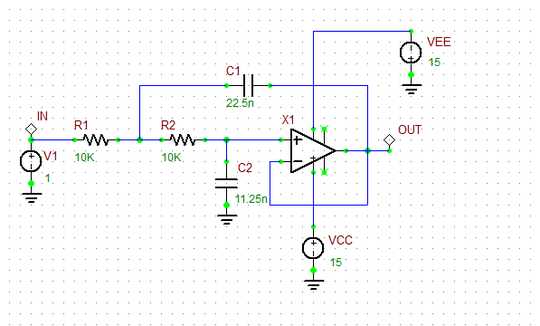The second-order active lowpass Sallen-Key filter.

To characterize your active filter, you will run an AC Sweep Test. Open the Test Panel of the Toolbox and define the start and stop frequencies of the AC frequency sweep as 1Hz and 50kHz, respectively. For this sweep, choose the "Decade" interval options with 50 Steps / Interval. Before you run your AC sweep, you will specify a custom plot for the simulation. This will be the gain of the filter defined as Vout / Vin expressed in the dB scale. For this purpose, click the "Preset Graph Plots..." button of the Test Panel to open up the "Edit Plot List" dialog. You will see that VDB(OUT) and VDB(IN) are already listed as the plots to be generated. Remove these plots from the list using the left arrow button. Instead, click the "Add Custom Plot" button to open up the "Edit Signal Plot" dialog. Define a new signal called "Gain" with the "Yellow" color and define as "VDB(OUT) - VDB(IN)" as shown in the figure below.

 Start Frequency 1Hz 50kHz 50 Decade Custom: Gain = VDB(OUT) - VDB(IN)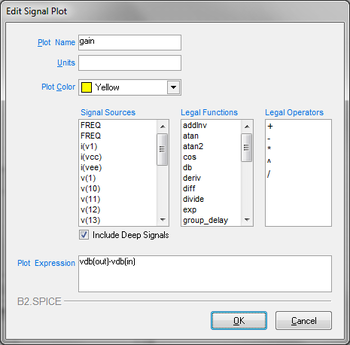Defining a custom plot in the Edit Signal Plot dialog.

Run an AC frequency sweep of your active filter. You should get a frequency response like the figure shown below. As you would have expected, the 3dB knee of the curve is located at 1kHz. From the graph you can see that at f = 5kHz, the gain drops to -28dB.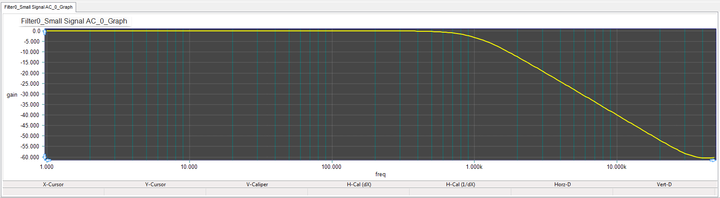The frequency response of the second-order active lowpass filter.

## Designing a Second-Order Active Highpass Filter

The following is a list of parts needed for this part of the tutorial lesson:

Part Name Part Type Part Value
VCC DC Voltage Source 15V
VEE DC Voltage Source -15V
V1 AC Voltage Source 1V
R1 Resistor 11.25k
R2 Resistor 22.5k
C1 Capacitor 10n
C2 Capacitor 10n
X1 LM741 Op-Amp Defaults

The basic second-order lowpass Sallen-Key active filter is shown in the above figure. The transfer function of this filter is given by:

$H(j\omega) = \frac{V_{out}} {V_{in}} = \frac {(j\omega)^2 R_1 R_2 C_1 C_2} {1-\omega ^2 R_1 R_2 C_1 C_2 + j\omega R_1 \left( C_1 + C_2 \right) }$

Setting s = jω, one can write:

$H(s) = \frac {s^2} {s^2 + s(\omega_0 / Q) + \omega_0 ^2} = \frac {s^2} { \left( s - s_1 \right) \left( s - s_2 \right) }$

where

$\omega_0 = \frac {1}{\sqrt{ R_1 R_2 C_1 C_2 } }$

$Q = \sqrt{\frac{R_2}{R_1}} \left( \frac{\sqrt{ C_1 C_2 }}{C_1 + C_2} \right)$

The same definitions of the poles s1 and s2 given earlier for the case of the lowpass filter are still valid for this case, too. The above expressions can be further simplified by setting C1 = C2 = C. Then we have:

$\omega_0 = \frac {1}{C \sqrt{ R_1 R_2 } }$

$Q = \frac{1}{2} \sqrt{\frac{R_2}{R_1}}$

To design a highpass filter with a cutoff frequency of f0 = ω0 / 2π = 1kHz and a maximally flat Q = 1/√2, and assuming C1 = C2 = C = 10nF, the above equations yield R2 = 2Q/(ω0C) = 22.5k and R1 = 1/(2Qω0C) = 11.25k. Place and connect the parts with the specified values as shown in the above figure. Run an AC frequency sweep of your active filter. You should get a frequency response like the figure shown below with a cutoff frequency of 1kHz.The second-order active highpass Sallen-Key filter.
 Start Frequency 1Hz 50kHz 50 Decade Custom: Gain = VDB(OUT) - VDB(IN)The frequency response of the second-order active highpass filter.

## Designing a Second-Order Active Bandpass Filter

The following is a list of parts needed for this part of the tutorial lesson:

Part Name Part Type Part Value
VCC DC Voltage Source 15V
VEE DC Voltage Source -15V
V1 AC Voltage Source 1V
R1 Resistor 11.25k
R2 Resistor 22.5k
C1 Capacitor 10n
C2 Capacitor 10n
X1 LM741 Op-Amp Defaults

The basic second-order bandpass Sallen-Key active filter is shown in the above figure. The transfer function of this filter is given by:

$H(j\omega) = \frac{V_{out}} {V_{in}} = \frac {(j\omega)^2 R_1 R_2 C_1 C_2} {1-\omega ^2 R_1 R_2 C_1 C_2 + j\omega R_1 \left( C_1 + C_2 \right) }$

Setting s = jω, one can write:

$H(s) = \frac {-\omega_0 ^2 R_2 C_2 s} {s^2 + s(\omega_0 / Q) + \omega_0 ^2} = \frac {-\omega_0 ^2 R_2 C_2 s} { \left( s - s_1 \right) \left( s - s_2 \right) }$

where

$\omega_0 = \frac {1}{\sqrt{ R_1 R_2 C_1 C_2 } }$

$Q = \sqrt{\frac{R_2}{R_1}} \left( \frac{\sqrt{ C_1 C_2 }}{C_1 + C_2} \right)$

The same definitions of the poles s1 and s2 given earlier for the case of the lowpass filter are still valid for this case, too. The above equations represent a biquad transfer function with one zero and two poles.

In particular, at the passband center frequency &omega0, we have:

$\left| H(j\omega_0) \right| = \left| \frac{V_{out}} {V_{in}} \right|_{\omega =\omega_0} = \frac {R_2 C_2} { R_1 \left( C_1 + C_2 \right) } = \sqrt{ \frac{R_2 C_2}{R_1 C_1} }Q$

The half-power bandwidth of the filter is defined as BW = ω2 - ω1. It can be shown that for large Q values, BW ≅ ω0 / Q. The above expressions can be further simplified by setting C1 = C2 = C. Then, you will get the same relationships as in the highpass case:

$\omega_0 = \frac {1}{C \sqrt{ R_1 R_2 } }$

$Q = \frac{1}{2} \sqrt{\frac{R_2}{R_1}}$

At the passband center frequency &omega0, you will have:

$\left| H(j\omega_0) \right| = \left| \frac{V_{out}} {V_{in}} \right|_{\omega =\omega_0} = \frac{1}{2} \frac {R_2} {R_1}$

In this part of the tutorial lesson, you will design a bandpass filter with a center frequency of f0 = ω0 / 2π = 1kHz and a maximally flat Q = 1/√2. Assuming C1 = C2 = C = 10nF, the above equations yield R2 = 2Q/(ω0C) = 22.5k and R1 = 1/(2Qω0R) = 11.25k . Place and connect the parts with the specified values as shown in the above figure. Run an AC frequency sweep of your active filter. But this time set the stop frequency of the sweep to 500kHz. You should get a frequency response like the figure shown below with a passband center frequency of 1kHz. Note that at 1kHz, the gain is 0dB because |H(jω0)| = R2/(2.R1) = (22.5k) / (2)(11.25k) = 1.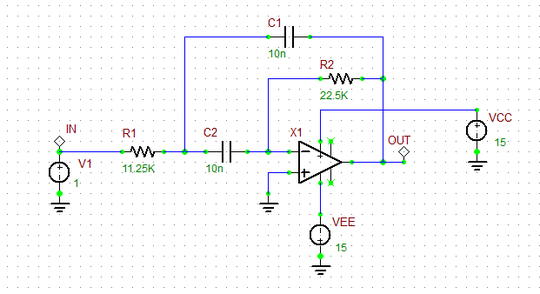The second-order active bandpass Sallen-Key filter.
 Start Frequency 1Hz 500kHz 50 Decade Custom: Gain = VDB(OUT) - VDB(IN)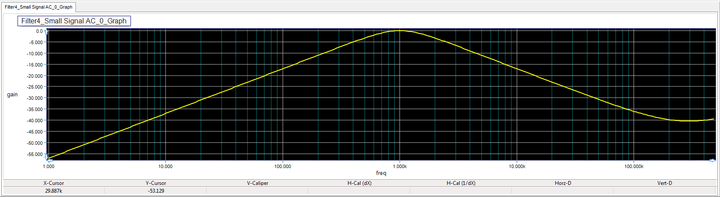The frequency response of the second-order active bandpass filter.

## Designing a Cascaded Fourth-Order Butterworth Lowpass Filter

The following is a list of parts needed for this part of the tutorial lesson:

Part Name Part Type Part Value
VCC DC Voltage Source 15V
VEE DC Voltage Source -15V
V1 AC Voltage Source 1V
R1 - R4 Resistor 10k
C1 Capacitor 17n
C2 Capacitor 15n
C3 Capacitor 42n
C4 Capacitor 6n
X1 - X2 LM741 Op-Amp Defaults

You can cascade two second-order lowpass filters to design a fourth-order lowpass filter as shown in the figure below. The transfer function of the cascaded systems is given by:

$H(s) = H_1(s) . H_2(s) = \frac {\omega_{01} ^2 \omega_{02} ^2 } { \left( s - s_1 \right) \left( s - s_2 \right) \left( s - s_3 \right) \left( s - s_4 \right) }$

The four poles of the fourth-order filter are nothing but the two pairs of poles of the constituent second-order lowpass stages. These poles can be determined according to the type of the filter response specification. For example, for the Butterworth filter, the poles are given by:

N = 4 Butterworth Poles
Pole 1 -0.924 + j 0.383
Pole 2 -0.924 - j 0.383
Pole 3 -0.383 + j 0.924
Pole 4 -0.383 - j 0.924

Based on the Butterworth poles, and assuming R1 = R2 = R3 = R4 = R = 10k, we can calculate the values of the four capacitors:

C1 = 17nF

C2 = 15nF

C3 = 42nF

C4 = 6nF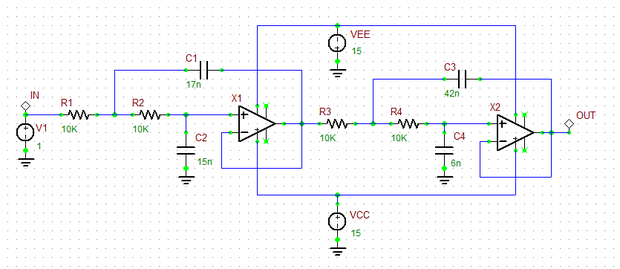The Fourth-Order Cascaded Active Bandpass Filter with Butterworth poles.

Place and connect the parts with the specified values as shown in the above figure. Run an AC frequency sweep of your active filter with the stop frequency of the sweep set to 50kHz. You should get a frequency response like the figure shown below with a cutoff frequency of 1kHz. You can see from the graph that frequency response of the fourth-order filter drops after the cutoff much faster and the value of gain at f = 5kHz is below -55dB.

 Start Frequency 1Hz 50kHz 50 Decade Custom: Gain = VDB(OUT) - VDB(IN)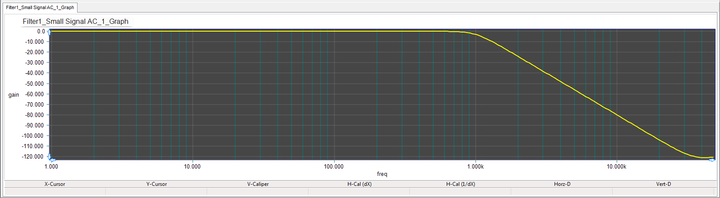The frequency response of the fourth-order active loswpass filter based on the Butterworth design.

## Designing a Cascaded Fourth-Order Chebyshev Lowpass Filter

The following is a list of parts needed for this part of the tutorial lesson:

Part Name Part Type Part Value
VCC DC Voltage Source 15V
VEE DC Voltage Source -15V
V1 AC Voltage Source 1V
R1 - R4 Resistor 10k
C1 Capacitor 187n
C2 Capacitor 1.5n
C3 Capacitor 77.2n
C4 Capacitor 16.7n
X1 - X2 LM741 Op-Amp Defaults

Next, we consider the Chebyshev filter design. With a ripple factor ε = 1, the maximum peak-to-peak ripples would be 3dB. For a fourth-order design, the poles are given by:

N = 4 Chebyshev Poles
Pole 1 -0.085 + j 0.947
Pole 2 -0.085 - j 0.947
Pole 3 -0.206 + j 0.392
Pole 4 -0.206 - j 0.392

Based on the 3-dB Chebyshev poles, and assuming R1 = R2 = R3 = R4 = R = 10k, we can calculate the values of the four capacitors:

C1 = 187nF

C2 = 1.5nF

C3 = 77.2nF

C4 = 16.7nF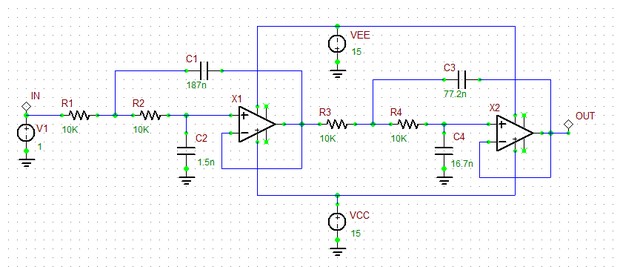The Fourth-Order Cascaded Active Bandpass Filter with Chebyshev poles.

Place and connect the parts with the specified values as shown in the above figure. Run an AC frequency sweep of your active filter with the stop frequency of the sweep set to 50kHz. You will get a frequency response like the figure shown below with a cutoff frequency of 1kHz. You can see from the graph that frequency response of this fourth-order filter drops even faster than the previous case and the value of gain at f = 5kHz is -70dB. However, now you have introduced a ripple in the frequency response with a maximum peak-to-peak amplitude of 3dB!

 Start Frequency 1Hz 50kHz 50 Decade Custom: Gain = VDB(OUT) - VDB(IN)The frequency response of the fourth-order active loswpass filter based on the 3dB Chebyshev design.